# How to Find Amplitude of a Sine Function

Lesson Transcript
Instructor: Gerald Lemay

Gerald has taught engineering, math and science and has a doctorate in electrical engineering.

In this lesson, we look at a method for finding the amplitude of a sine function. This method works even when the equilibrium line is not the horizontal axis. Updated: 04/20/2021

## Finding the Amplitude

In general, we can write a sine function as: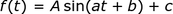The function of time, f(t), equals the amplitude, A, times the sine of at plus b, plus a vertical offset, c. If we're given an equation of this form, the amplitude of the sine function is simply A. When we don't have the equation and only a plot, we have to be careful. The vertical offset, c, can cause some difficulty in finding the amplitude.

The amplitude is defined as the vertical distance from the equilibrium line to the maximum of the curve, with the the maximum of the curve being called the crest. This vertical distance is the same as the distance from the equilibrium line to the minimum of the curve, with the minimum of the curve being called the trough. If there is no vertical offset, as in c = 0, the equilibrium line is the horizontal axis and the crest equals the amplitude.

When the equilibrium line isn't the horizontal axis, we can't say the amplitude is the crest. We can either find the new equilibrium line or we can use the peak values of the sine function. In this lesson we will use the peak values to determine the amplitude.

By the way, a is the frequency in radians per second (often written as ω) and b is related to the horizontal shift. With t as the independent variable, we can find the horizontal shift by setting at + b equal to 0 and solving for t. Then, the horizontal shift equals -b/a. This horizontal shift is usually called the phase shift.

A sinusoid with arbitrary phase shift and frequency looks like this here: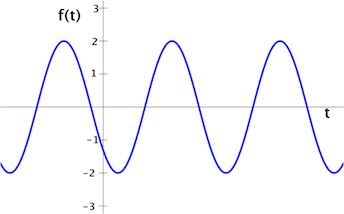The steps for finding the amplitude are as follows:

#### Step 1: Determine the maximum and minimum vertical displacements

We can draw horizontal lines locating these displacements.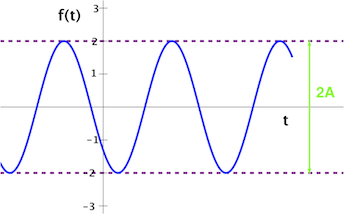The maximum vertical displacement (the crest) is 2. The minimum vertical displacement (the trough) is -2.

#### Step 2: Take the difference of max minus min and divide by 2

max - min = 2 - (-2) = 4 and 4 divided by 2 is 2. Thus, the amplitude, A is 2.

In this example, the vertical offset, c, was zero.

#### The Final Result

The amplitude, A, of a sine function is given by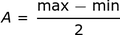An error occurred trying to load this video.

Try refreshing the page, or contact customer support.

Coming up next: How to Find the Area of an Equilateral Triangle

### You're on a roll. Keep up the good work!

Replay
Your next lesson will play in 10 seconds
• 0:03 Finding the Amplitude
• 2:30 Non-zero Vertical Offset
• 3:41 Lesson Summary
Save Save

Want to watch this again later?

Timeline
Autoplay
Autoplay
Speed Speed

## Non-Zero Vertical Offset

These steps will work even if there's a vertical offset. For example, let's find the amplitude, A, for the following graph: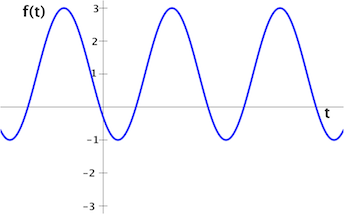#### Step 1: Determine the maximum and minimum vertical displacements

The maximum vertical displacement is 3, while the minimum vertical displacement is -1.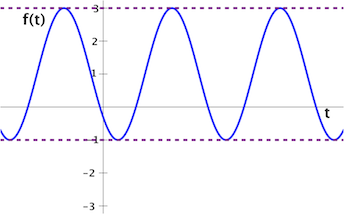To unlock this lesson you must be a Study.com Member.

### Register to view this lesson

Are you a student or a teacher?